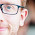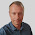## Wednesday, July 29, 2015

### Simple volatility rebalancing strategy

In my posts, I often mention a volatility rebalancing strategy. Originally, this strategy supposed to rebalance a portfolio daily to local volatility measured by standard deviation. I strongly suspect, that a common retail investor will stop trying to understand this strategy right after words "standard deviation", so I propose a simplified version of this strategy.

My purpose was to replace standard deviation as a volatility measure with something more simple. And since daily rebalancing may be too cumbersome for an investor, I wanted to make sure that changing a rebalancing frequency from daily to weekly will not harm results.

I found, that it is possible to replace a monthly standard deviation with a 15-day price channel width. Correlation between these two metrics is 0.87 in case of SPY. Changing rebalancing frequency from daily to weekly doesn't make Sharpe ratio worse.

So here is the simplified version of volatility rebalancing strategy. Assuming we have N stocks in our portfolio, every Friday around the market close we do the following calculations.

- Dividing our capital value C on N parts to get how much capital we have for one stock.

- Dividing the result on share price P to get a number of shares we would have to buy if we wanted a simple equal-weighted allocation.

- Now adjusting this number of shares, multiplying it on K = 6% / ((H / L - 1) * 100%), where H and L are highest and lowest prices inside a 15-day (including today) price channel. That 6% is an average price channel width for the SPY, so the average adjustment ratio will be around 1 in case of SPY.

So, the number of shares is: V = (C / (N * P)) * 6% / ((H / L - 1) * 100%)

For example, compare these two equities for the SPY without rebalancing (red) and with rebalancing (black), dividends are reinvested:

Sharpe ratio is 0.46 without rebalancing and 0.59 with rebalancing. Expenses are not included here.

1.1.